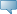## Catalog Entries

Current
Feb 22, 2020Select the Course Number to get further detail on the course. Select the desired Schedule Type to find available classes for the course.

 MAT 320 - Number Theory Number Theory Prequisite: MAT 230 This course covers a range of ideas from number theory, starting with the division algorithm, modular arithmetic, and divisibility tests. Further topics include the extended Euclidean algorithm, the Fundamental Theorem of Arithmetic, the Chinese Remainder Theorem, Wilson's Theorem, Fermat's Little Theorem, the Euler phi-function, the existence of primitive roots, the theory of indices, and the study of the group integers mod n. Students will be required to write formal proofs. 3.000 Credit hours 3.000 Lecture hours Levels: Undergraduate Schedule Types: Lecture Mathematical Sciences Department